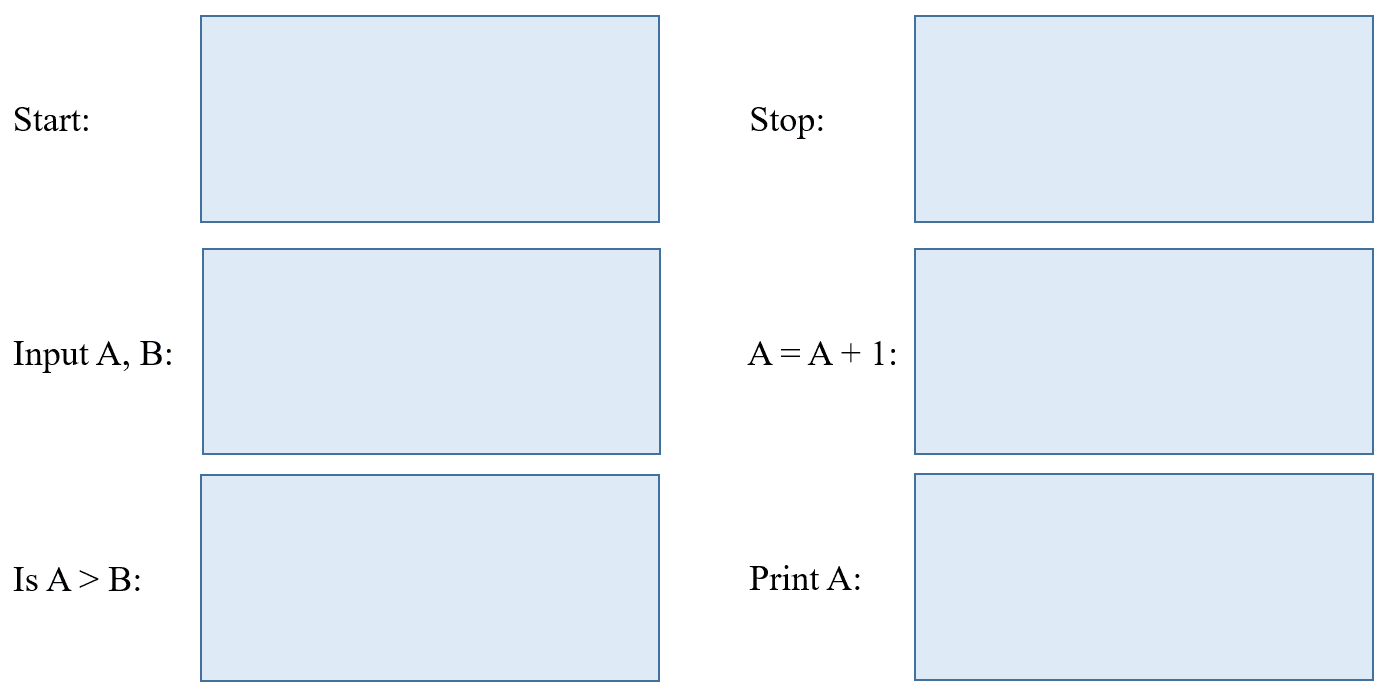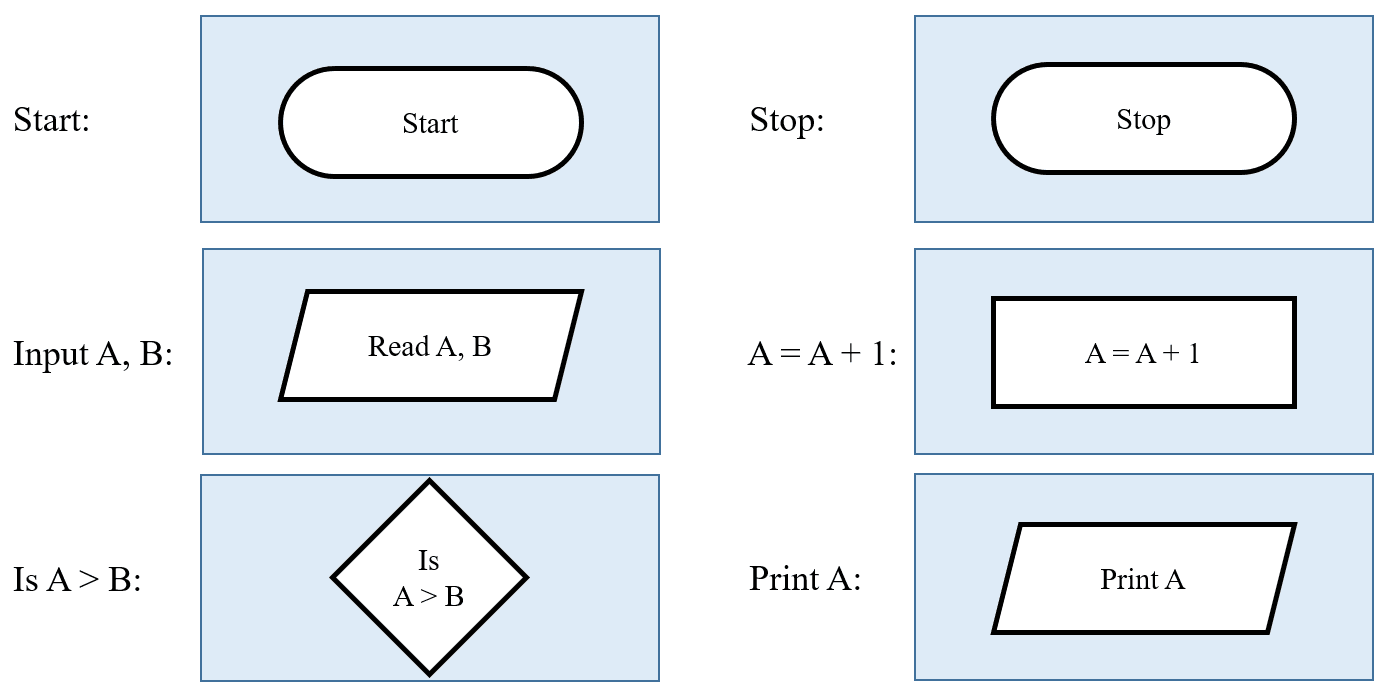# vs.eyeandcontacts.com

## Chapter 10 Algorithm and Flowchart

Brain Developer
A. Fill in the blanks:
1. An algorithm is a set of sequential steps that are developed in solving any problem.
2. The start/stop box symbol represents the starting or ending point of a program.
3. The processing box is used for writing the processing instructions.
4. The input/output box is used to display input and output of a program.
5. The decision box represents all comparisons and decisions.
6. The connectors are used to connect one part of flowchart to another.

B. State True or False:
1. A flowchart is not a pictorial representation of steps to get the solution of a problem. (False)
A flowchart is a pictorial representation of steps to get the solution of a problem.
2. Algorithm means a set of rules which specify how to solve some problems.  (True)
3. Flowcharts are helpful in analyzing the logic of problems. (True)
4. Connectors are used to connect the boxes. (True)
5. The general direction of flow in any flowchart is from bottom to top or right to left. (False)
The general direction of flow in any flowchart is from top to bottom or left to right.

C. Draw the flowchart boxes for the following:D. Multiple Choice Questions:
1. Algorithm is always written in ___________ language.
a) Difficult
b) Simple
c) Binary
b) Simple

2. _______________ box is used to display the final result.
a) Input/Output
b) Stop
c) Processing
a) Input/Output

3. In a flowchart, _________________ indicates the sequence of steps and direction of flow.
a) Start/Stop box
b) Connectors
c) Flow lines
c) Flow lines

4. The diagrammatic representation that illustrates the steps for the solution of a problem is called _______________.
a) Algorithm
b) Flowchart
c) None
b) Flowchart

E. Answer the following:
1. Define the term flowchart.
A flowchart is a pictorial representation of steps or an algorithm used for solving a particular problem.

2. What is an Algorithm?
Algorithm is a set of sequential steps to solve any logical or a mathematical problem. An algorithm is written in a simple language.

3. What is the use of Input/Output box?
Input/Output box is used for accepting input or giving output of the program.

4. When do we use the Process box?
Process box is used for writing the processing instructions and doing calculations.

5. What is the use of the Decision box?
Decision box is used for checking or applying any condition in the program.

6. Write the difference between Flowchart and Algorithm.